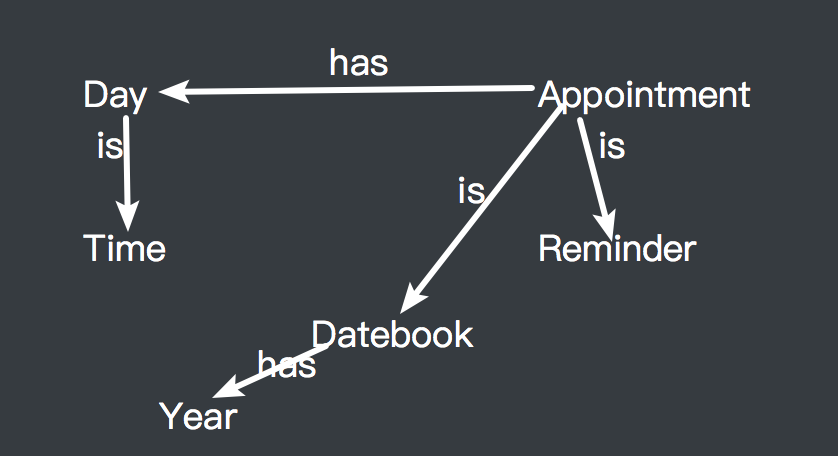# Gym 101673E Is-A? Has-A? Who Knowz-A?（搜索）

## Is-A? Has-A? Who Knowz-A?

Two familiar concepts in object oriented programming are the is-a and has-a relationships. Given two classes A and B, we say that A is-a B if A is a subclass of B; we say A has-a B if one of the fields of A is of type B. For example, we could imagine an object-oriented language (call it ICPC++) with code like that in Figure 1, where the class Day is-a Time, the class Appointment is both a DateBook and a Reminder, and class Appointment has-a Day.

 class Day extends Time    class Appointment extends Datebook, Reminder
{                         {
...                       private Day date;
}                            ...
}

These two relationships are transitive. For example if A is-a B and B is-a C then it follows that A is-a C. This holds as well if we change all the is-a’s in the last sentence to has-a’s. It also works with combinations of is-a’s and has-a’s: in the example above, Appointment has-a Time, since it has-a Day and Day is-a Time. Similarly, if class DateBook has-a Year then Appointment has-a Year, since Appointment is-a DateBook.

In this problem you will be given a set of is-a and has-a relationships and a set of queries of the form A is/has-a B. You must determine if each query is true or false.

### Input:

Input starts with two integers nn and mm, (1≤n,m≤10000), where nn specifies the number of given is-a and has-a relationships and mm specifies the number of queries. The next nn lines each contain one given relationship in the form c1 r c2 where c1 and c2 are single-word class names, and r is either the string “is-a” or “has-a”. Following this are mm queries, one per line, using the same format. There will be at most 500 distinct class names in the n+m lines, and all class names in the last mm lines will appear at least once in the initial nn lines. All is-a and has-a relationships between the given classes can be deduced from the nn given relationships. Is-a relationships can not be circular (apart from the trivial identity “xx is-a xx”).

### Output

For each query, display the query number (starting at one) and whether the query is true or false.

### Sample Input 1

5 5
Day is-a Time
Appointment is-a Datebook
Appointment is-a Reminder
Appointment has-a Day
Datebook has-a Year
Day is-a Time
Time is-a Day
Appointment has-a Time
Appointment has-a Year
Day is-a Day

### Sample Output 1

Query 1: true
Query 2: false
Query 3: true
Query 4: true
Query 5: true

### 链接

https://cn.vjudge.net/problem/Gym-101673E

### 题意

• $is \rightarrow is = is$
• $has \rightarrow has = has$
• $has \rightarrow is = has$
• $is \rightarrow has = has$

### 题解### 代码

StatusAccepted
Time421ms
Memory2720kB
Length1969
#include <iostream>
#include <cstdio>
#include <algorithm>
#include <cstring>
#include <map>
#include <queue>
#define pii pair<int, int>
#define ff first
#define ss second
using namespace std;
const int maxn = 510;
bool vis[maxn][maxn]; // 0表示is关系，1表示has关系
int is[maxn][maxn];
int has[maxn][maxn];
map <string, int> mp;
int tot = 0;

int strint(string s) // 字符串转化为数字标号
{
if (mp.count(s))
return mp[s];
mp[s] = tot++;
}

void bfs(int u)
{
queue <pii> Q;
vis[u][u] = 1;
Q.push(pii(u, 0)); // 节点与is/has关系
while (!Q.empty())
{
pii v = Q.front();
Q.pop();
for (int i = 0; i < tot; ++i)
{
if (!vis[u][i][v.ss] && is[v.ff][i]) // is-is -> is
{
vis[u][i][v.ss] = 1;
Q.push(pii(i, v.ss));
}
if (!vis[u][i] && has[v.ff][i]) // is-has -> has / has-is -> has / has-has -> has
{
vis[u][i] = 1;
Q.push(pii(i, 1));
}
}
}
}

int main()
{
int n, m;
string a, b, c;
scanf("%d%d", &n, &m);
mp.clear();
memset(vis, 0, sizeof(vis));
for (int i = 0; i < n; ++i)
{
cin >> a >> b >> c;
strint(a), strint(c);
if (b == 'i') // is关系建边
is[strint(a)][strint(c)] = 1;
else if (b == 'h') // has关系建边
has[strint(a)][strint(c)] = 1;
}
for (int i = 0; i < tot; ++i) // 对每个节点进行搜索改变相应关系
bfs(i);
for (int i = 1; i <= m; ++i)
{
cin >> a >> b >> c;
if (b == 'i')
{
if (vis[strint(a)][strint(c)])
printf("Query %d: true\n", i);
else
printf("Query %d: false\n", i);
}
else
{
if (vis[strint(a)][strint(c)])
printf("Query %d: true\n", i);
else
printf("Query %d: false\n", i);
}
}
return 0;
}

The end.
2018-08-16 星期四